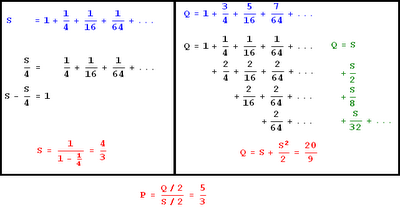## Wednesday, January 6, 2010

### A simple infinite series

By request, I will show you how to calculate the following:

P = (1/2 + 3/8 + 5/32 + 7/128 + ...) / (1/2 + 1/8 + 1/32 + 1/128 + ...)

Let Q/2 be the numerator and S/2 be the denominator. Here is a visual solution:And if you like calculus, here's an alternate way to calculate Q:I guess now my blog will greet new readers with a wall of math for the next day or so.

#### 1 comment:Secret Squïrrel said...

There's a simpler formula for convergent series if the first term is 1. You have to identify a single number to multiply each successive term by which requires you to chop Q up as you have done.

Call this multiplier "M".
Sum = 1 / (1-M)

S = 1 / (1-1/4)
S = 1 / (3/4)
S = 1.33

Works for the meta-summing of Q too.